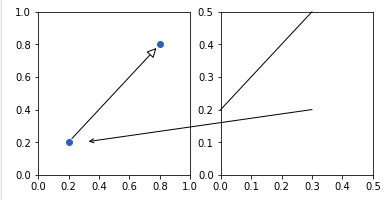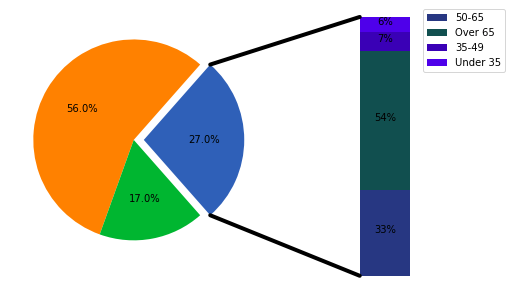# Matplotlib.patches.ConnectionPatch class in Python

Matplotlib is an amazing visualization library in Python for 2D plots of arrays. Matplotlib is a multi-platform data visualization library built on NumPy arrays and designed to work with the broader SciPy stack.

## matplottlib.patches.ConnectionPatch

The `matplottlib.patches.ConnectionPatch `a subclass of `matplotlib.patches.FancyArrowPatch` class and are used for making connecting lines between two points.

Syntax: class matplotlib.patches.ConnectionPatch(xyA, xyB, coordsA, coordsB=None, axesA=None, axesB=None, arrowstyle=’-‘, arrow_transmuter=None, connectionstyle=’arc3’, connector=None, patchA=None, patchB=None, shrinkA=0.0, shrinkB=0.0, mutation_scale=10.0, mutation_aspect=None, clip_on=False, dpi_cor=1.0, **kwargs)

Parameters:

• xyA: It is the start point of connecting line on x-y plot also called Point A.
• xyB: It is the start point of connecting line on x-y plot also called Point B.
• coordsA: Coordinate of point A.
• coordsB: Coordinate of point B.
• axesA: It is the start point of connecting axes on x-y plot.
• axesB: It is the end point of connecting axes on x-y plot.
• arrowstyle: It is used for styling the connection arrow. Its default type is ‘-‘.
• arrow_transmuter: It is used to ignore a connecting line.
• connectionstyle: It describes how posA and posB are connected. It can be an instance of the class ConnectionStyle or a string by the name of connectionstyle, it has optional comma-separated attributes.
• connector: It is generally ignored and decides which connector to ignore.
• patchA: Used to add patches at point A.
• patchB: Used to add patches at point B
• shrinkA: Used to shrink the connector at point A.
• shrinkB: Used to shrink the connector at point B.
• mutation_scale: Value with which attributes of arrowstyle (e.g., head_length) gets scaled.
• mutation_aspect: The height of the rectangle will be squeezed by this value before the mutation and the mutated box will be stretched by the inverse of it.
• clip_on: Set whether the artist uses clipping.
• dpi_cor: dpi_cor is currently used for linewidth-related things and shrink factor. Mutation scale is affected by this.

The below are list of valid Kwargs key;

Key Description
arrowstyle the arrow style
connectionstyle the connection style
relpos default is (0.5, 0.5)
patchA default is bounding box of the text
patchB default is None
shrinkA default is 2 points
shrinkB default is 2 points
mutation_scale default is text size (in points)
mutation_aspect default is 1.
? any key for` matplotlib.patches.PathPatch`

The coordinates of xyA and xyB are indicated by a string coordsA and coordsB.

Property Description
‘figure points’ points from the lower left corner of the figure
‘figure pixels’ pixels from the lower left corner of the figure
‘figure fraction’ 0, 0 is lower left of figure and 1, 1 is upper right
‘axes points’ points from lower left corner of axes
‘axes pixels’ pixels from lower left corner of axes
‘axes fraction’ 0, 0 is lower left of axes and 1, 1 is upper right
‘data’ use the coordinate system of the object being annotated (default)
‘offset points’ offset (in points) from the xy value
‘polar’ you can specify theta, r for the annotation, even in cartesian plots. Note that if you are using a polar axes, you do not need to specify polar for the coordinate system since that is the native “data” coordinate system.

Example 1:

 `from` `matplotlib.patches ``import` `ConnectionPatch ` `import` `matplotlib.pyplot as plt ` ` `  `fig, (ax1, ax2) ``=` `plt.subplots(``1``, ``2``,  ` `                               ``figsize ``=``(``6``, ``3``)) ` ` `  `# Draw a simple arrow between  ` `# two points in axes coordinates ` `# within a single axes. ` `xyA ``=` `(``0.2``, ``0.2``) ` `xyB ``=` `(``0.8``, ``0.8``) ` `coordsA ``=` `"data"` `coordsB ``=` `"data"` `con ``=` `ConnectionPatch(xyA, xyB, ` `                      ``coordsA, coordsB, ` `                      ``arrowstyle ``=``"-|>"``, ` `                      ``shrinkA ``=` `5``, shrinkB ``=` `5``, ` `                      ``mutation_scale ``=` `20``,  ` `                      ``fc ``=``"w"``) ` ` `  `ax1.plot([xyA[``0``], xyB[``0``]], [xyA[``1``], ` `                            ``xyB[``1``]], ``"o"``) ` `ax1.add_artist(con) ` ` `  `# Draw an arrow between the  ` `# same point in data coordinates, ` `# but in different axes. ` `xy ``=` `(``0.3``, ``0.2``) ` `con ``=` `ConnectionPatch( ` `    ``xyA ``=` `xy, coordsA ``=` `ax2.transData, ` `    ``xyB ``=` `xy, coordsB ``=` `ax1.transData, ` `    ``arrowstyle ``=``"->"``, shrinkB ``=` `5``) ` ` `  `ax2.add_artist(con) ` ` `  `# Draw a line between the different ` `# points, defined in different coordinate ` `# systems. ` `con ``=` `ConnectionPatch( ` `    ``# in axes coordinates ` `    ``xyA ``=``(``0.6``, ``1.0``), coordsA ``=` `ax2.transAxes, ` `    ``# x in axes coordinates, y in data coordinates ` `    ``xyB ``=``(``0.0``, ``0.2``), coordsB ``=` `ax2.get_yaxis_transform(), ` `    ``arrowstyle ``=``"-"``) ` ` `  `ax2.add_artist(con) ` ` `  `ax1.set_xlim(``0``, ``1``) ` `ax1.set_ylim(``0``, ``1``) ` `ax2.set_xlim(``0``, .``5``) ` `ax2.set_ylim(``0``, .``5``) ` ` `  `plt.show() `

Output:Example 2:

 `import` `matplotlib.pyplot as plt ` `from` `matplotlib.patches ``import` `ConnectionPatch ` `import` `numpy as np ` ` `  ` `  `# make figure and assign axis  ` `# objects ` `fig ``=` `plt.figure(figsize ``=``(``9``, ``5``)) ` `ax1 ``=` `fig.add_subplot(``121``) ` `ax2 ``=` `fig.add_subplot(``122``) ` `fig.subplots_adjust(wspace ``=` `0``) ` ` `  `# pie chart parameters ` `ratios ``=` `[.``27``, .``56``, .``17``] ` `explode ``=` `[``0.1``, ``0``, ``0``] ` ` `  `# rotate so that first wedge is ` `# split by the x-axis ` `angle ``=` `-``180` `*` `ratios[``0``] ` `ax1.pie(ratios, autopct ``=``'% 1.1f %%'``, ` `        ``startangle ``=` `angle, ` `        ``explode ``=` `explode) ` ` `  `# bar chart parameters ` ` `  `xpos ``=` `0` `bottom ``=` `0` `ratios ``=` `[.``33``, .``54``, .``07``, .``06``] ` `width ``=` `.``2` `colors ``=` `[[.``1``, .``3``, .``5``], ` `          ``[.``1``, .``3``, .``3``], ` `          ``[.``1``, .``3``, .``7``], ` `          ``[.``1``, .``3``, .``9``]] ` ` `  `for` `j ``in` `range``(``len``(ratios)): ` `    ``height ``=` `ratios[j] ` `    ``ax2.bar(xpos, height, width,  ` `            ``bottom ``=` `bottom, ` `            ``color ``=` `colors[j]) ` `     `  `    ``ypos ``=` `bottom ``+` `ax2.patches[j].get_height() ``/` `2` `    ``bottom ``+``=` `height ` `    ``ax2.text(xpos,  ` `             ``ypos, ` `             ``"% d %%"` `%` `(ax2.patches[j].get_height() ``*` `100``), ` `             ``ha ``=``'center'``) ` ` `  `ax2.set_title('') ` `ax2.legend((``'50-65'``, ``'Over 65'``, ``'35-49'``, ``'Under 35'``)) ` `ax2.axis(``'off'``) ` `ax2.set_xlim(``-` `2.5` `*` `width, ``2.5` `*` `width) ` ` `  `# use ConnectionPatch to draw ` `# lines between the two plots ` `# get the wedge data ` `theta1, theta2 ``=` `ax1.patches[``0``].theta1, ax1.patches[``0``].theta2 ` `center, r ``=` `ax1.patches[``0``].center, ax1.patches[``0``].r ` `bar_height ``=` `sum``([item.get_height() ``for` `item ``in` `ax2.patches]) ` ` `  `# draw top connecting line ` `x ``=` `r ``*` `np.cos(np.pi ``/` `180` `*` `theta2) ``+` `center[``0``] ` `y ``=` `np.sin(np.pi ``/` `180` `*` `theta2) ``+` `center[``1``] ` `con ``=` `ConnectionPatch(xyA ``=``(``-``width ``/` `2``, bar_height), ` `                      ``coordsA ``=` `ax2.transData, ` `                      ``xyB ``=``(x, y),  ` `                      ``coordsB ``=` `ax1.transData) ` ` `  `con.set_color([``0``, ``0``, ``0``]) ` `con.set_linewidth(``4``) ` `ax2.add_artist(con) ` ` `  `# draw bottom connecting line ` `x ``=` `r ``*` `np.cos(np.pi ``/` `180` `*` `theta1) ``+` `center[``0``] ` `y ``=` `np.sin(np.pi ``/` `180` `*` `theta1) ``+` `center[``1``] ` ` `  `con ``=` `ConnectionPatch(xyA ``=``(``-``width ``/` `2``, ``0``),  ` `                      ``coordsA ``=` `ax2.transData, ` `                      ``xyB ``=``(x, y),  ` `                      ``coordsB ``=` `ax1.transData) ` ` `  `con.set_color([``0``, ``0``, ``0``]) ` `ax2.add_artist(con) ` `con.set_linewidth(``4``) ` ` `  `plt.show() `

Output:My Personal Notes arrow_drop_upCheck out this Author's contributed articles.

If you like GeeksforGeeks and would like to contribute, you can also write an article using contribute.geeksforgeeks.org or mail your article to contribute@geeksforgeeks.org. See your article appearing on the GeeksforGeeks main page and help other Geeks.

Please Improve this article if you find anything incorrect by clicking on the "Improve Article" button below.

Article Tags :

Be the First to upvote.

Please write to us at contribute@geeksforgeeks.org to report any issue with the above content.